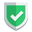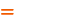我们相信：世界是美好的，你是我也是。平行空间的世界里面，不同版本的生活也在继续...

## 基本逻辑描述

``````class test {
public \$b;
public function __construct(\$v) {
\$this->b = \$v;
}
}
\$b1 = [["初始值a", "b", "c"], new test("初始值b")];
// ...
// 这个位置将会出现一系列的数组clone方案，但是其中有些是正确的，有些是错误的。
// ...
\$b1 = "修改值aa";
\$b1->b = "修改值bb";
// ...
// 一系列数组clone方案中，到底谁对谁错呢？
// ...``````
``````b1
Array
(
 => Array
(
 => 修改值aa
 => b
 => c
)

 => test Object
(
[b] => 修改值bb
)
)``````

## 正确的方案

``````\$b2 = array_copy(\$b1);
\$b3 = unserialize(serialize(\$b1));
\$b4 = json_decode(json_encode(\$b1));``````

newsn.net：这里是【评论】可见内容

## 错误的方案

``````\$b5 = \$b1;
\$b6 = array_merge([], \$b1);
\$b7 = (array)\$b1;
\$b8 = new ArrayObject(\$b1);
\$b9 = array_map(function (\$object) {
return clone \$object;
}, \$b1);``````

newsn.net：这里是【评论】可见内容

## 结论如果本文对您有帮助，或者节约了您的时间，欢迎打赏瓶饮料，建立下友谊关系。本博客不欢迎：各种镜像采集行为。请尊重原创文章内容，转载请保留作者链接。【福利】 腾讯云最新爆款活动！1核2G云服务器首年50元！【源码】本文代码片段及相关软件，请点此获取更多信息【绝密】秘籍文章入口，仅传授于有缘之人php